Скачать презентацию WSAC 2010 Talk 11 st January 2010 Cook-Levin

43c0439e88e98d1883d8f7e27a532aff.ppt

• Количество слайдов: 49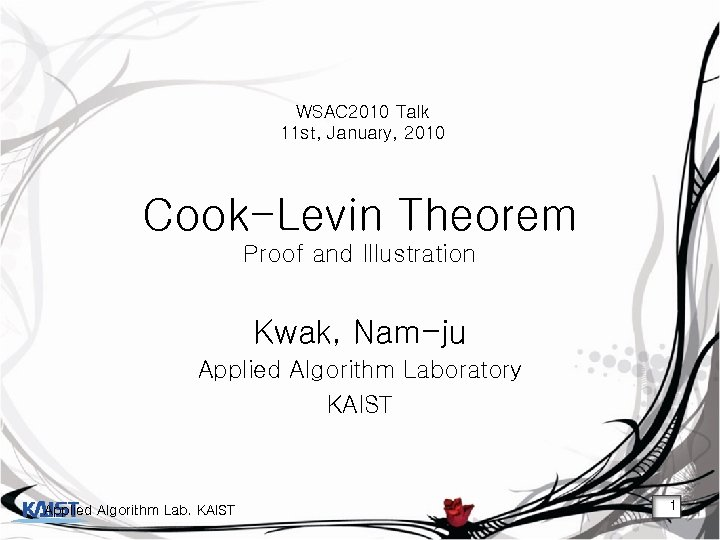WSAC 2010 Talk 11 st, January, 2010 Cook-Levin Theorem Proof and Illustration Kwak, Nam-ju Applied Algorithm Laboratory KAIST Applied Algorithm Lab. KAIST 1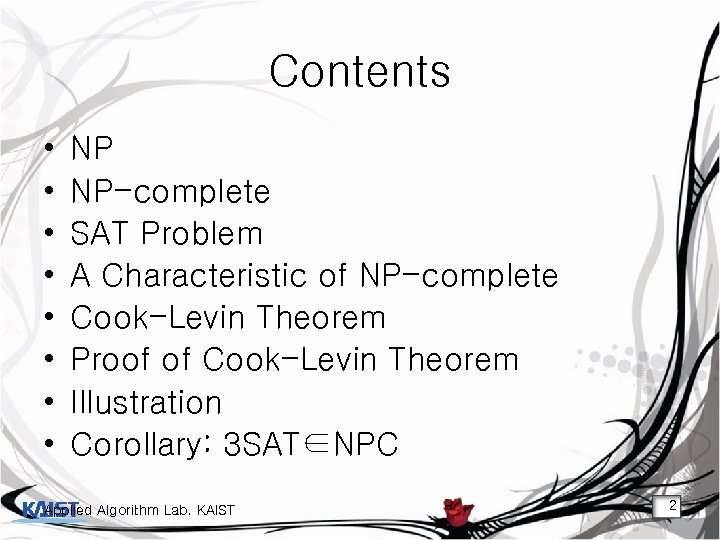Contents • • NP NP-complete SAT Problem A Characteristic of NP-complete Cook-Levin Theorem Proof of Cook-Levin Theorem Illustration Corollary: 3 SAT∈NPC Applied Algorithm Lab. KAIST 2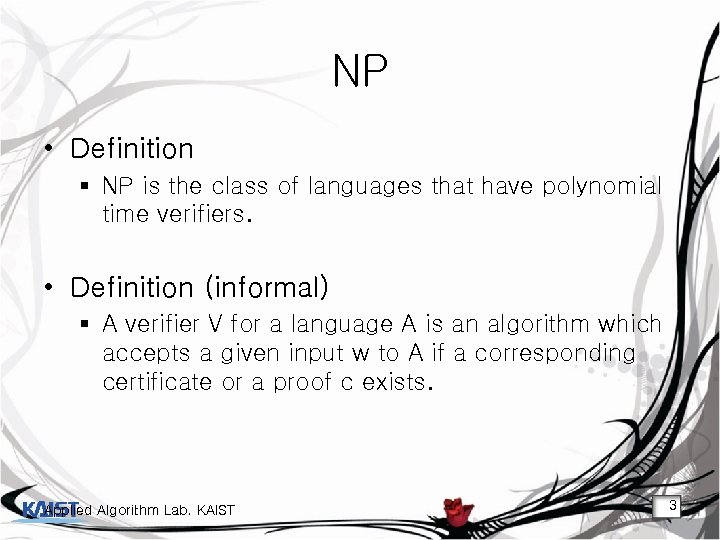NP • Definition § NP is the class of languages that have polynomial time verifiers. • Definition (informal) § A verifier V for a language A is an algorithm which accepts a given input w to A if a corresponding certificate or a proof c exists. Applied Algorithm Lab. KAIST 3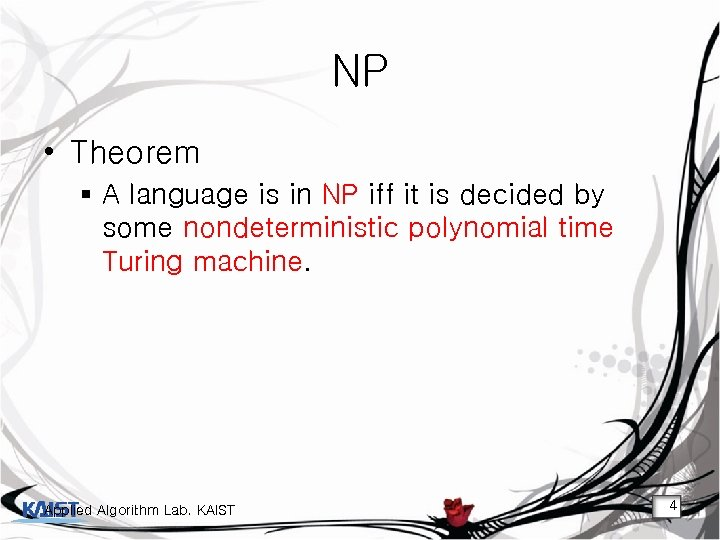NP • Theorem § A language is in NP iff it is decided by some nondeterministic polynomial time Turing machine. Applied Algorithm Lab. KAIST 4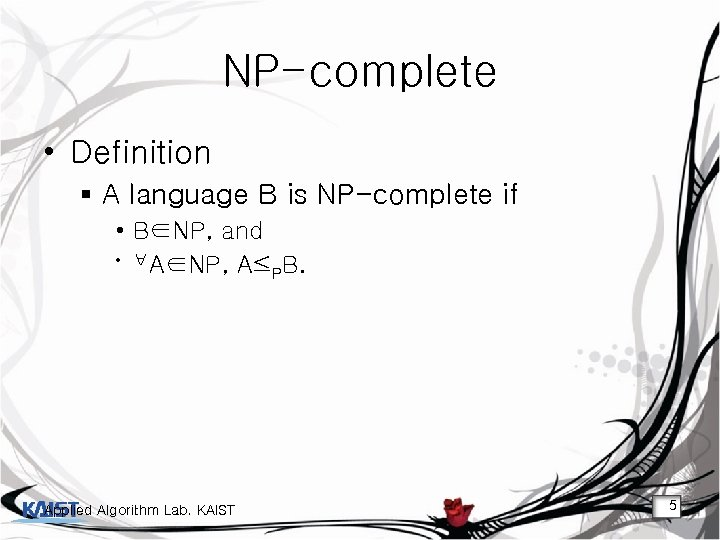NP-complete • Definition § A language B is NP-complete if • B∈NP, and • ∀A∈NP, A≤ B. P Applied Algorithm Lab. KAIST 5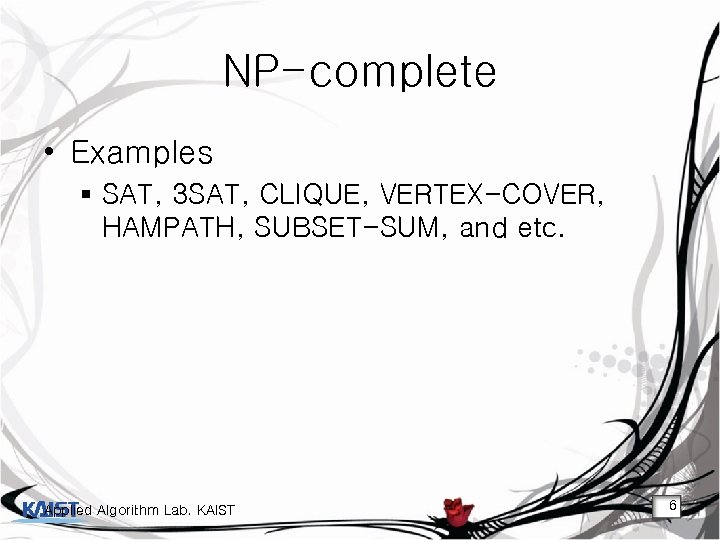NP-complete • Examples § SAT, 3 SAT, CLIQUE, VERTEX-COVER, HAMPATH, SUBSET-SUM, and etc. Applied Algorithm Lab. KAIST 6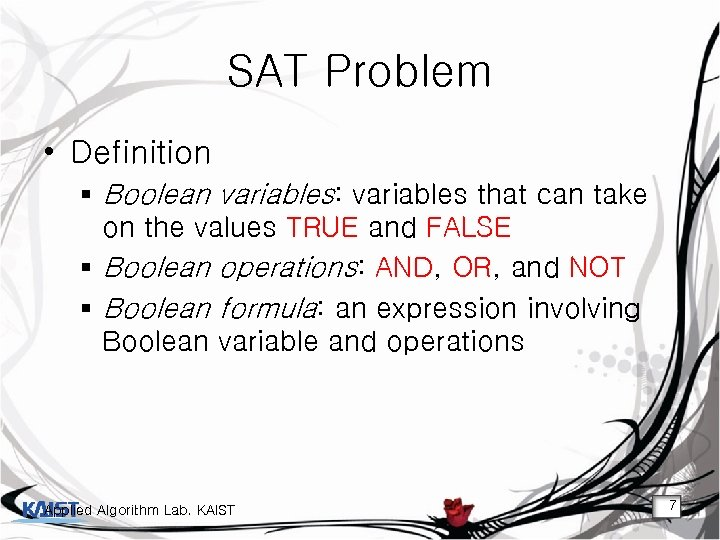SAT Problem • Definition § Boolean variables: variables that can take on the values TRUE and FALSE § Boolean operations: AND, OR, and NOT § Boolean formula: an expression involving Boolean variable and operations Applied Algorithm Lab. KAIST 7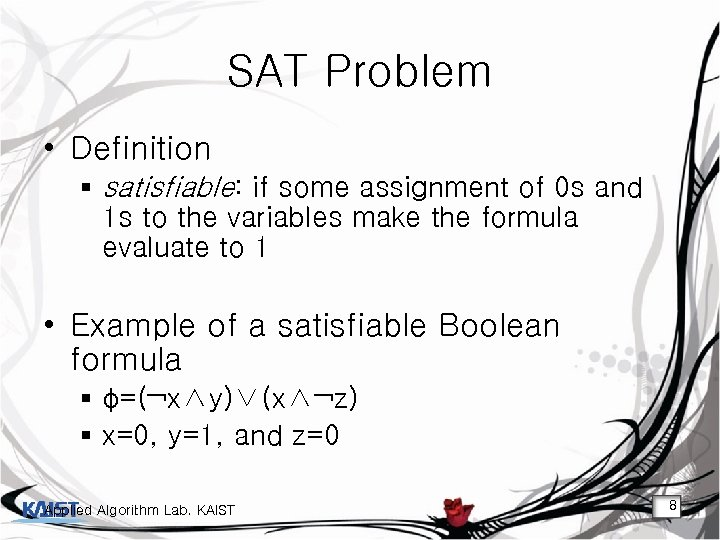SAT Problem • Definition § satisfiable: if some assignment of 0 s and 1 s to the variables make the formula evaluate to 1 • Example of a satisfiable Boolean formula § φ=(¬x∧y)∨(x∧¬z) § x=0, y=1, and z=0 Applied Algorithm Lab. KAIST 8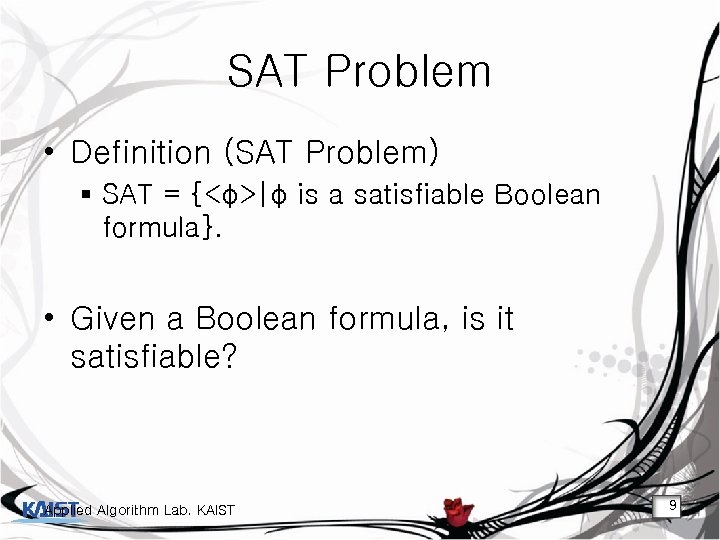SAT Problem • Definition (SAT Problem) § SAT = {<φ>|φ is a satisfiable Boolean formula}. • Given a Boolean formula, is it satisfiable? Applied Algorithm Lab. KAIST 9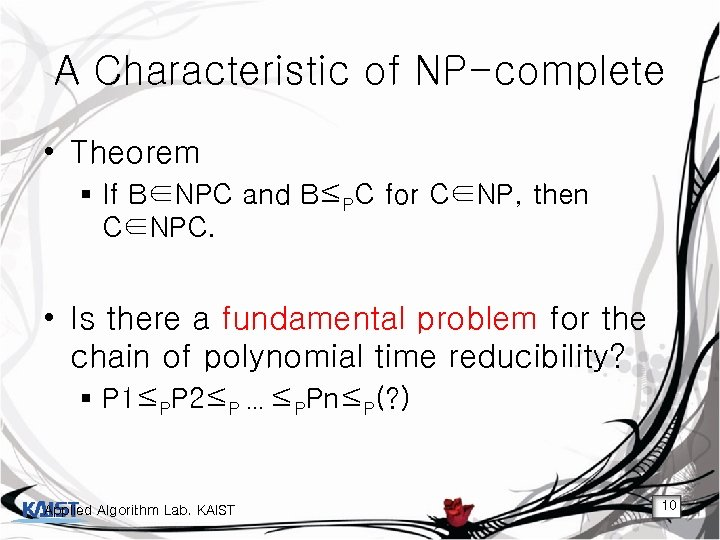A Characteristic of NP-complete • Theorem § If B∈NPC and B≤PC for C∈NP, then C∈NPC. • Is there a fundamental problem for the chain of polynomial time reducibility? § P 1≤PP 2≤P … ≤PPn≤P(? ) Applied Algorithm Lab. KAIST 10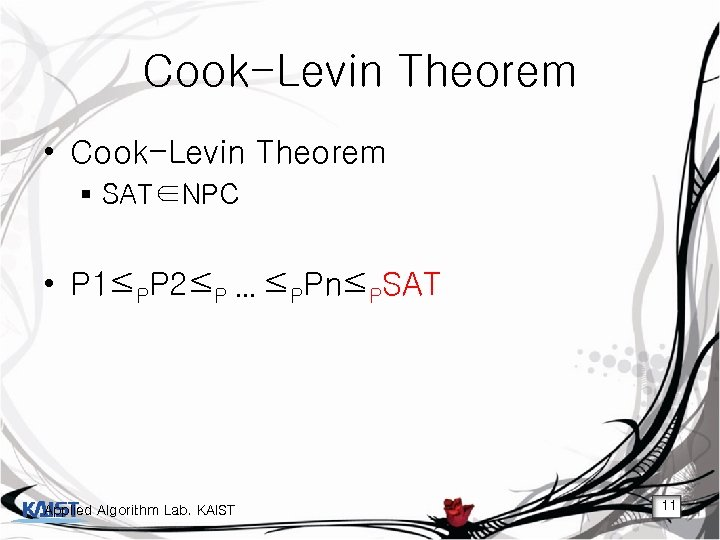Cook-Levin Theorem • Cook-Levin Theorem § SAT∈NPC • P 1≤PP 2≤P … ≤PPn≤PSAT Applied Algorithm Lab. KAIST 11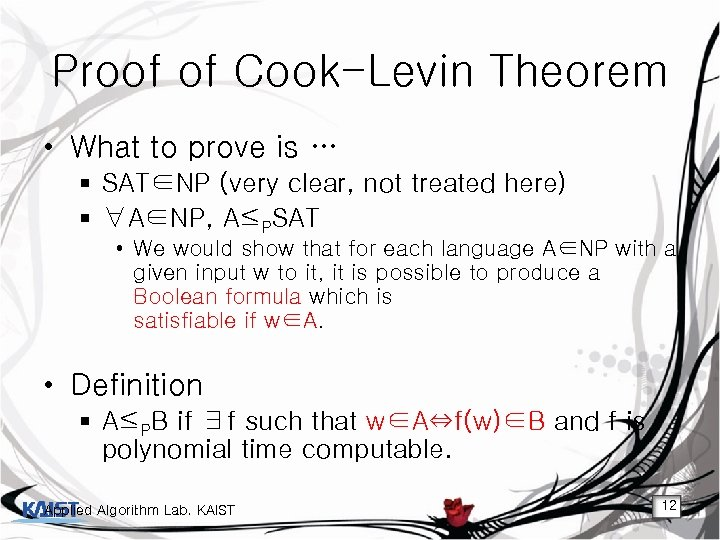Proof of Cook-Levin Theorem • What to prove is … § SAT∈NP (very clear, not treated here) § ∀A∈NP, A≤PSAT • We would show that for each language A∈NP with a given input w to it, it is possible to produce a Boolean formula which is satisfiable if w∈A. • Definition § A≤PB if ∃f such that w∈A⇔f(w)∈B and f is polynomial time computable. Applied Algorithm Lab. KAIST 12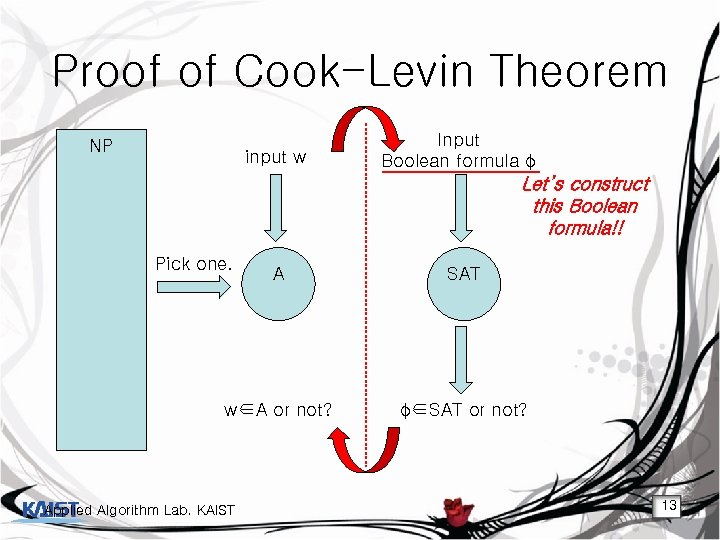Proof of Cook-Levin Theorem NP input w Input Boolean formula φ Let’s construct this Boolean formula!! Pick one. A SAT w∈A or not? φ∈SAT or not? Applied Algorithm Lab. KAIST 13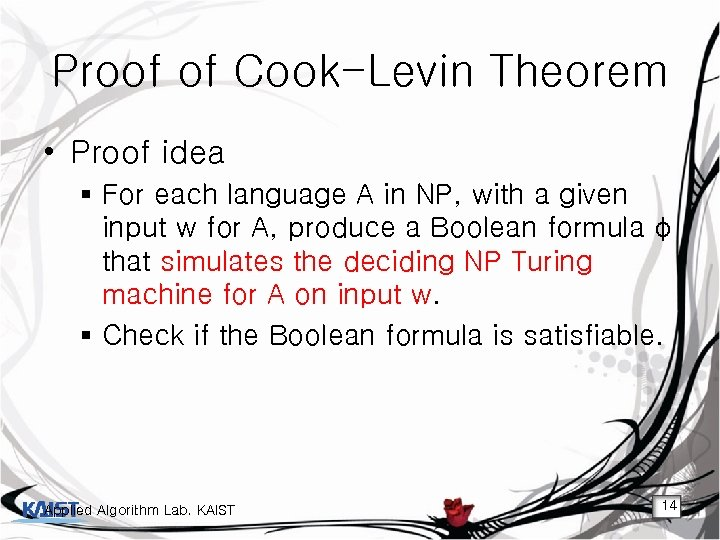Proof of Cook-Levin Theorem • Proof idea § For each language A in NP, with a given input w for A, produce a Boolean formula φ that simulates the deciding NP Turing machine for A on input w. § Check if the Boolean formula is satisfiable. Applied Algorithm Lab. KAIST 14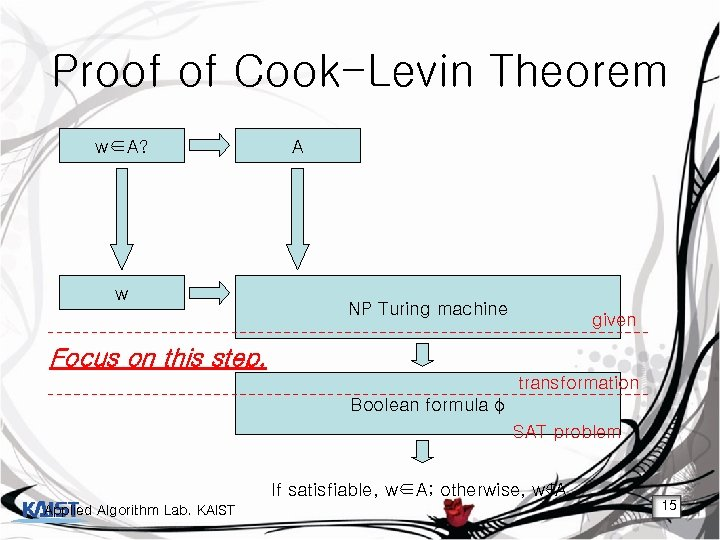Proof of Cook-Levin Theorem w∈A? w A NP Turing machine given Focus on this step. transformation Boolean formula φ SAT problem If satisfiable, w∈A; otherwise, w∉A. Applied Algorithm Lab. KAIST 15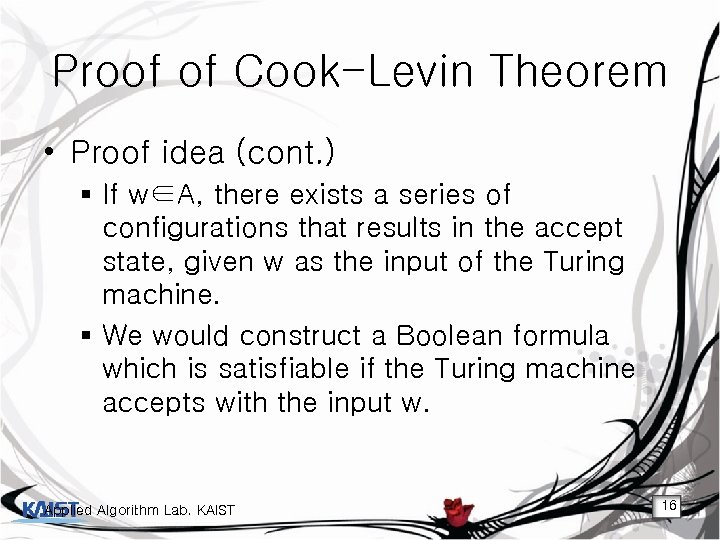Proof of Cook-Levin Theorem • Proof idea (cont. ) § If w∈A, there exists a series of configurations that results in the accept state, given w as the input of the Turing machine. § We would construct a Boolean formula which is satisfiable if the Turing machine accepts with the input w. Applied Algorithm Lab. KAIST 16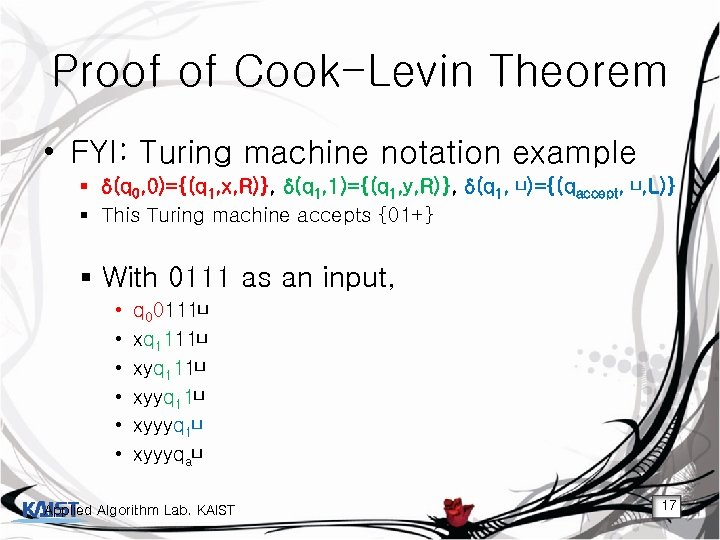Proof of Cook-Levin Theorem • FYI: Turing machine notation example § δ(q 0, 0)={(q 1, x, R)}, δ(q 1, 1)={(q 1, y, R)}, δ(q 1, ⊔)={(qaccept, ⊔, L)} § This Turing machine accepts {01+} § With 0111 as an input, • • • q 00111⊔ xq 1111⊔ xyq 111⊔ xyyq 11⊔ xyyyqa⊔ Applied Algorithm Lab. KAIST 17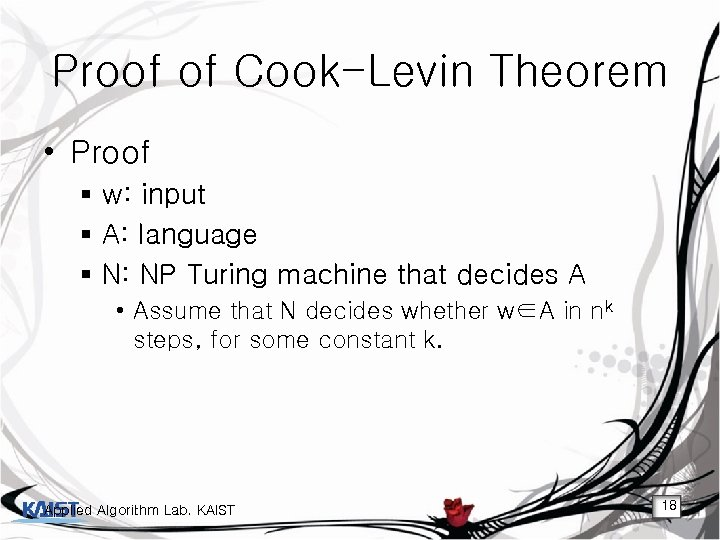Proof of Cook-Levin Theorem • Proof § w: input § A: language § N: NP Turing machine that decides A • Assume that N decides whether w∈A in nk steps, for some constant k. Applied Algorithm Lab. KAIST 18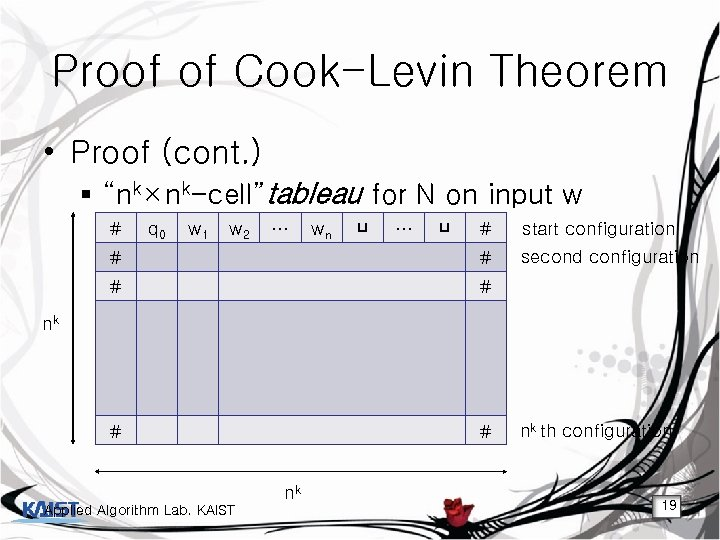Proof of Cook-Levin Theorem • Proof (cont. ) § “nk×nk-cell”tableau for N on input w # q 0 w 1 w 2 … wn ⊔ … ⊔ # start configuration # # second configuration # # nk nk Applied Algorithm Lab. KAIST nk th configuration 19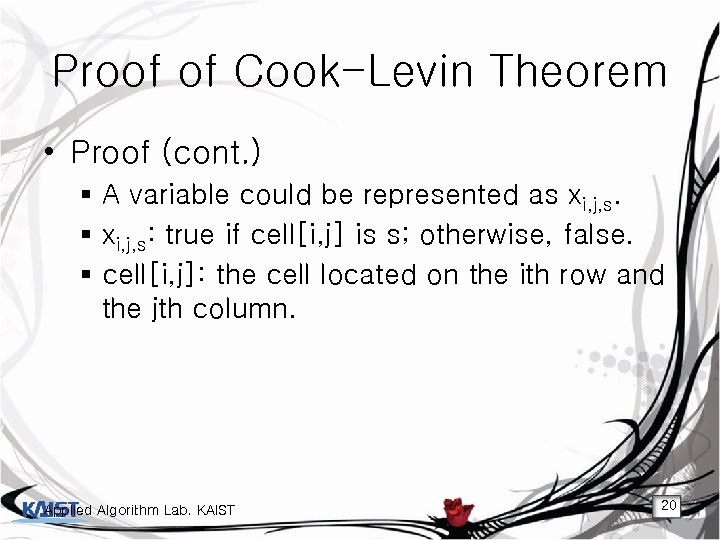Proof of Cook-Levin Theorem • Proof (cont. ) § A variable could be represented as xi, j, s. § xi, j, s: true if cell[i, j] is s; otherwise, false. § cell[i, j]: the cell located on the ith row and the jth column. Applied Algorithm Lab. KAIST 20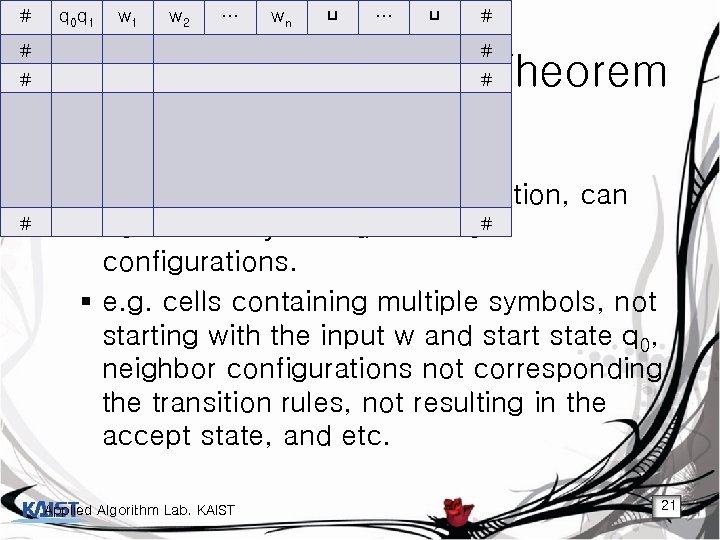# # q 00 q 1 w 1 w 1 q 3 w 2 x q w 2 … wn … ⊔ ⊔ # # x wn ⊔ … ⊔ # x q 1 q 2 w 1 … … # ⊔ # # x x q Proof … w Cook-Levin #Theorem of ⊔ … ⊔ # 1 n # ⊔ ⊔ ⊔ … ⊔ # # # • Proof (cont. ) § The tableau, without any restriction, can # # contain many invalid series of configurations. § e. g. cells containing multiple symbols, not starting with the input w and start state q 0, neighbor configurations not corresponding the transition rules, not resulting in the accept state, and etc. Applied Algorithm Lab. KAIST 21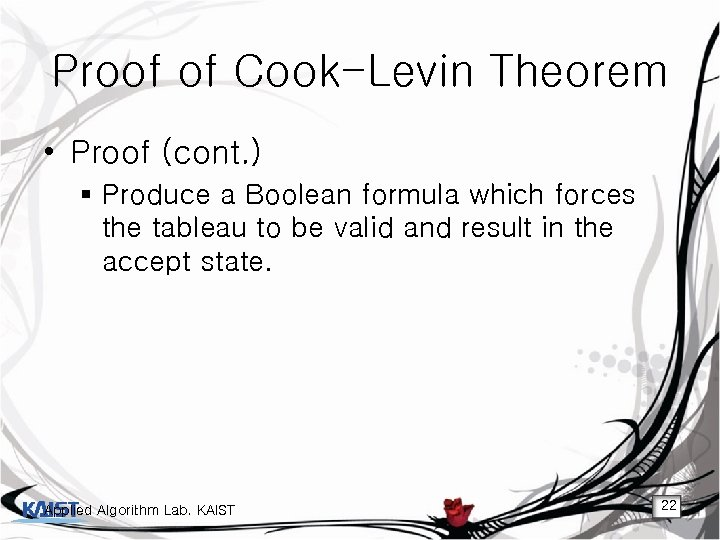Proof of Cook-Levin Theorem • Proof (cont. ) § Produce a Boolean formula which forces the tableau to be valid and result in the accept state. Applied Algorithm Lab. KAIST 22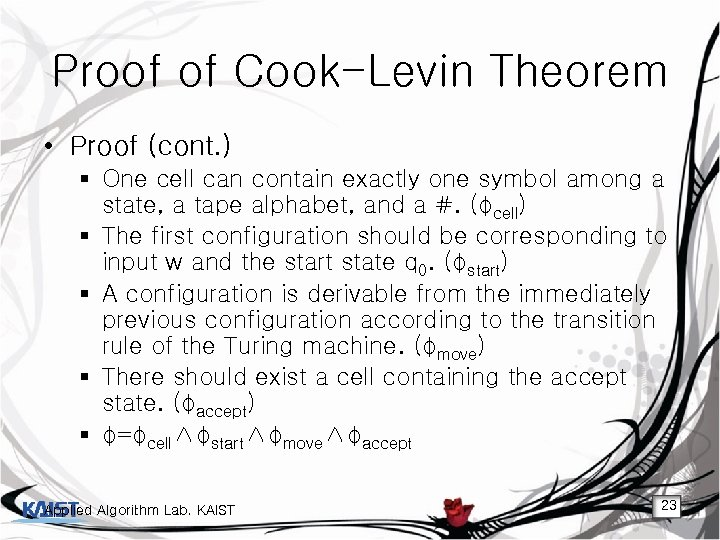Proof of Cook-Levin Theorem • Proof (cont. ) § One cell can contain exactly one symbol among a state, a tape alphabet, and a #. (φcell) § The first configuration should be corresponding to input w and the start state q 0. (φstart) § A configuration is derivable from the immediately previous configuration according to the transition rule of the Turing machine. (φmove) § There should exist a cell containing the accept state. (φaccept) § φ=φcell∧φstart∧φmove∧φaccept Applied Algorithm Lab. KAIST 23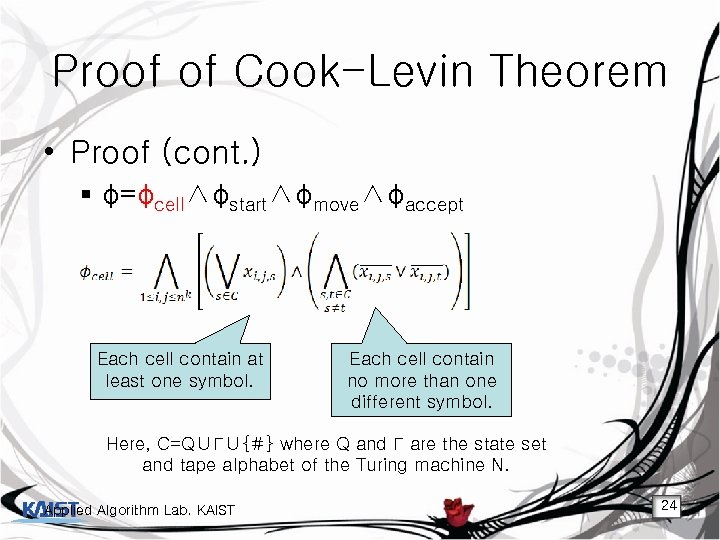Proof of Cook-Levin Theorem • Proof (cont. ) § φ=φcell∧φstart∧φmove∧φaccept Each cell contain at least one symbol. Each cell contain no more than one different symbol. Here, C=Q∪Γ∪{#} where Q and Γ are the state set and tape alphabet of the Turing machine N. Applied Algorithm Lab. KAIST 24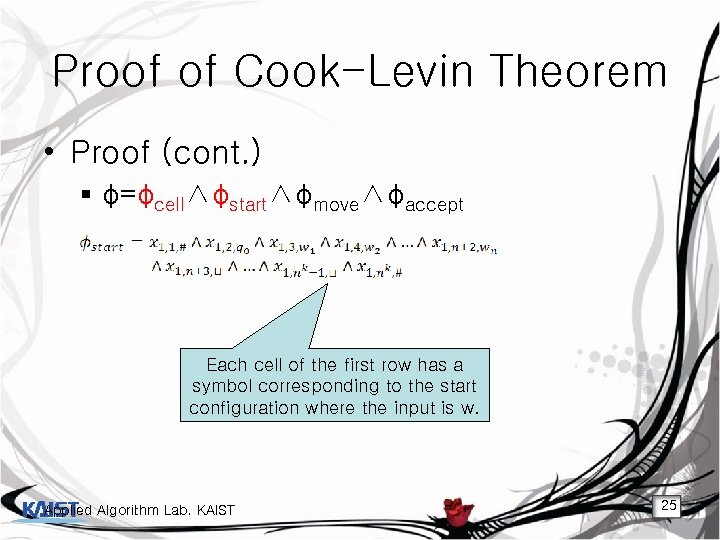Proof of Cook-Levin Theorem • Proof (cont. ) § φ=φcell∧φstart∧φmove∧φaccept Each cell of the first row has a symbol corresponding to the start configuration where the input is w. Applied Algorithm Lab. KAIST 25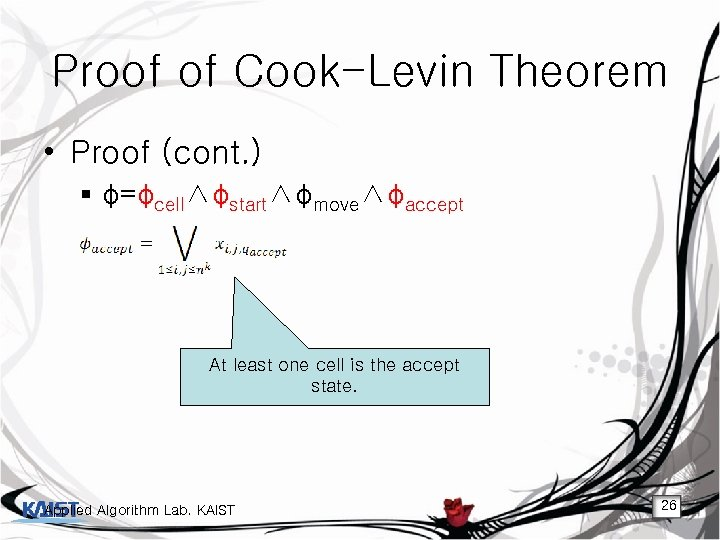Proof of Cook-Levin Theorem • Proof (cont. ) § φ=φcell∧φstart∧φmove∧φaccept At least one cell is the accept state. Applied Algorithm Lab. KAIST 26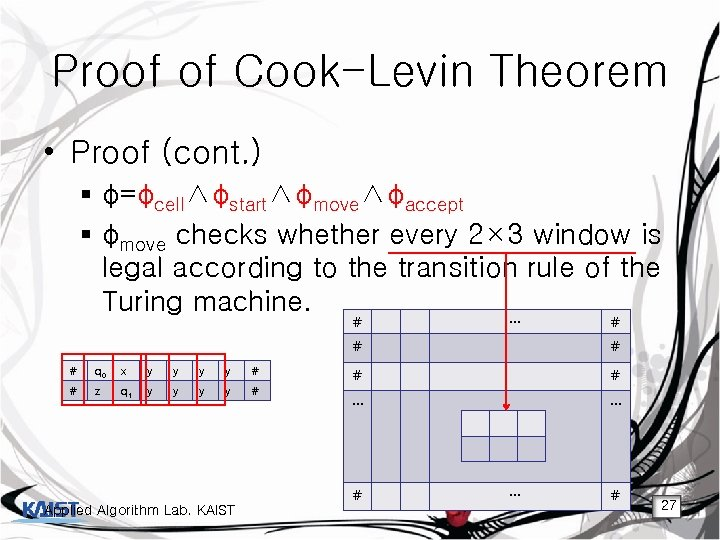Proof of Cook-Levin Theorem • Proof (cont. ) § φ=φcell∧φstart∧φmove∧φaccept § φmove checks whether every 2× 3 window is legal according to the transition rule of the Turing machine. # … # # # q 0 x y y # # z q 1 y y # # # … … # # Applied Algorithm Lab. KAIST … # 27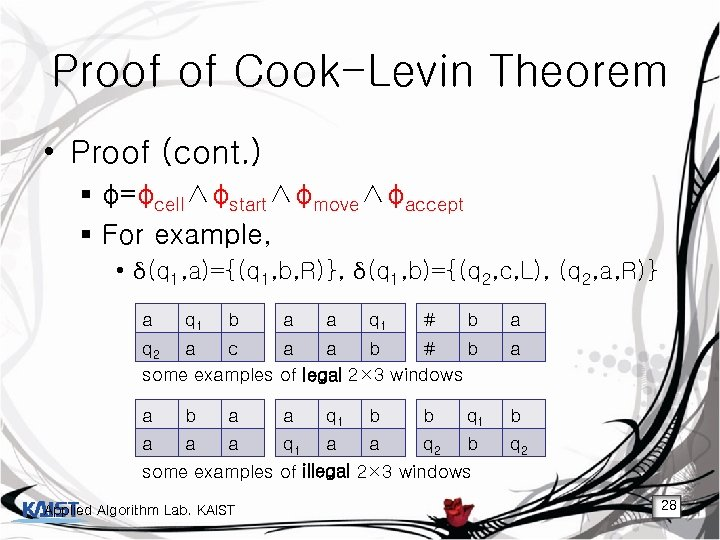Proof of Cook-Levin Theorem • Proof (cont. ) § φ=φcell∧φstart∧φmove∧φaccept § For example, • δ(q 1, a)={(q 1, b, R)}, δ(q 1, b)={(q 2, c, L), (q 2, a, R)} a q 1 b a a q 1 # b a q 2 a c a a b # b some examples of legal 2× 3 windows a a b a a q 1 b b q 1 b a a a q 1 a a q 2 b q 2 some examples of illegal 2× 3 windows Applied Algorithm Lab. KAIST 28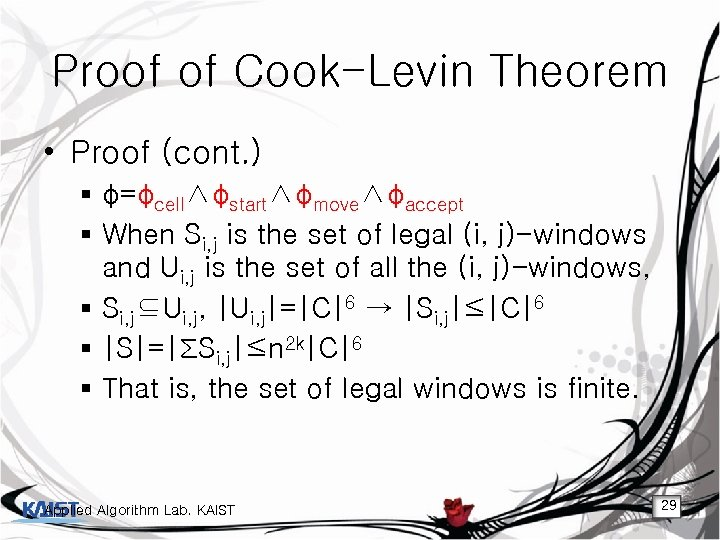Proof of Cook-Levin Theorem • Proof (cont. ) § φ=φcell∧φstart∧φmove∧φaccept § When Si, j is the set of legal (i, j)-windows and Ui, j is the set of all the (i, j)-windows, § Si, j⊆Ui, j, |Ui, j|=|C|6 → |Si, j|≤|C|6 § |S|=|ΣSi, j|≤n 2 k|C|6 § That is, the set of legal windows is finite. Applied Algorithm Lab. KAIST 29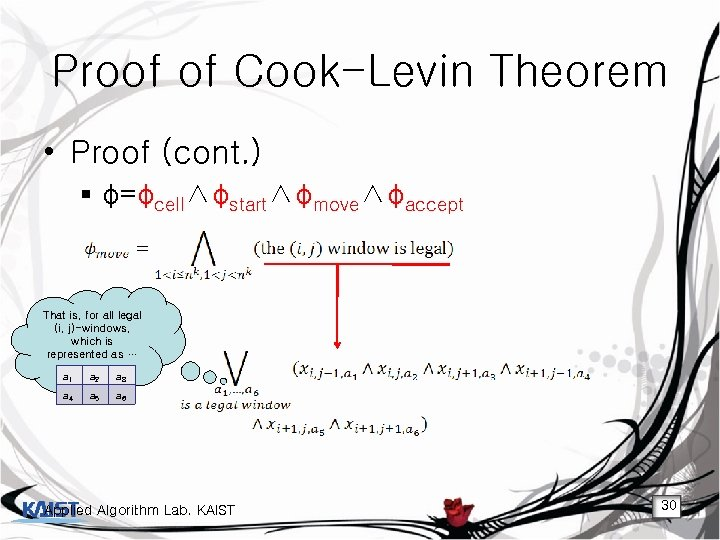Proof of Cook-Levin Theorem • Proof (cont. ) § φ=φcell∧φstart∧φmove∧φaccept That is, for all legal (i, j)-windows, which is represented as … a 1 a 2 a 3 a 4 a 5 a 6 Applied Algorithm Lab. KAIST 30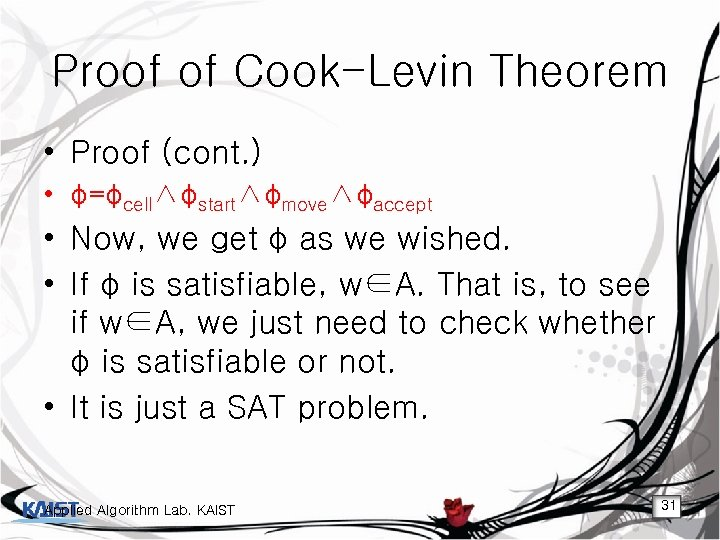Proof of Cook-Levin Theorem • Proof (cont. ) • φ=φcell∧φstart∧φmove∧φaccept • Now, we get φ as we wished. • If φ is satisfiable, w∈A. That is, to see if w∈A, we just need to check whether φ is satisfiable or not. • It is just a SAT problem. Applied Algorithm Lab. KAIST 31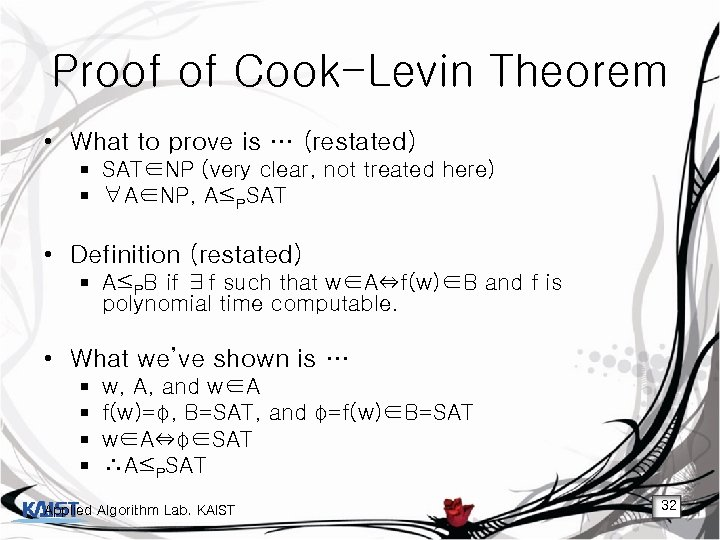Proof of Cook-Levin Theorem • What to prove is … (restated) § SAT∈NP (very clear, not treated here) § ∀A∈NP, A≤PSAT • Definition (restated) § A≤PB if ∃f such that w∈A⇔f(w)∈B and f is polynomial time computable. • What we’ve shown is … § § w, A, and w∈A f(w)=φ, B=SAT, and φ=f(w)∈B=SAT w∈A⇔φ∈SAT ∴A≤PSAT Applied Algorithm Lab. KAIST 32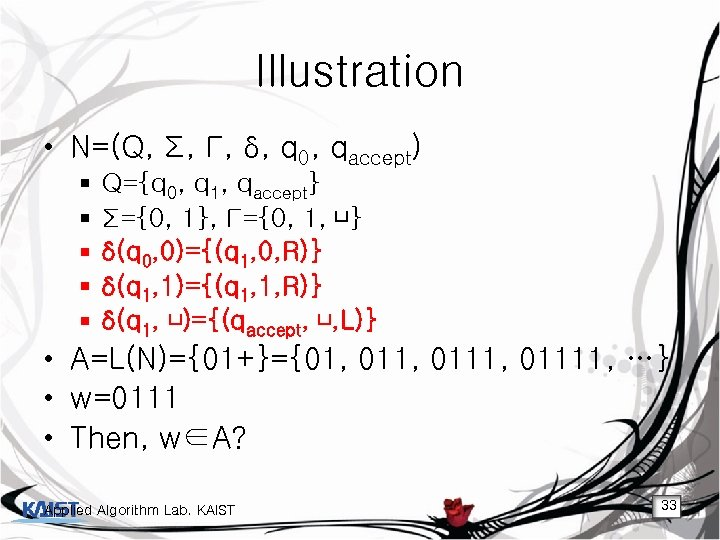Illustration • N=(Q, Σ, Γ, δ, q 0, qaccept) § § § Q={q 0, q 1, qaccept} Σ={0, 1}, Γ={0, 1, ⊔} δ(q 0, 0)={(q 1, 0, R)} δ(q 1, 1)={(q 1, 1, R)} δ(q 1, ⊔)={(qaccept, ⊔, L)} • A=L(N)={01+}={01, 0111, 01111, …} • w=0111 • Then, w∈A? Applied Algorithm Lab. KAIST 33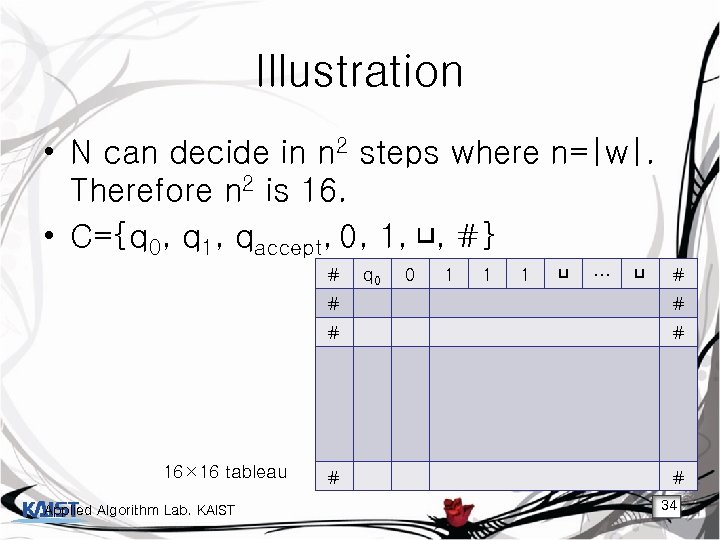Illustration • N can decide in n 2 steps where n=|w|. Therefore n 2 is 16. • C={q 0, q 1, qaccept, 0, 1, ⊔, #} # q 0 0 1 1 1 ⊔ … ⊔ # # # 16× 16 tableau Applied Algorithm Lab. KAIST # # 34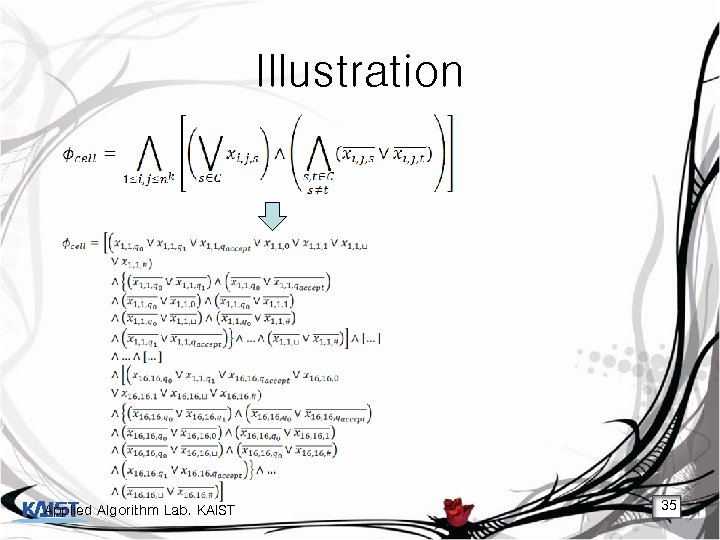Illustration Applied Algorithm Lab. KAIST 35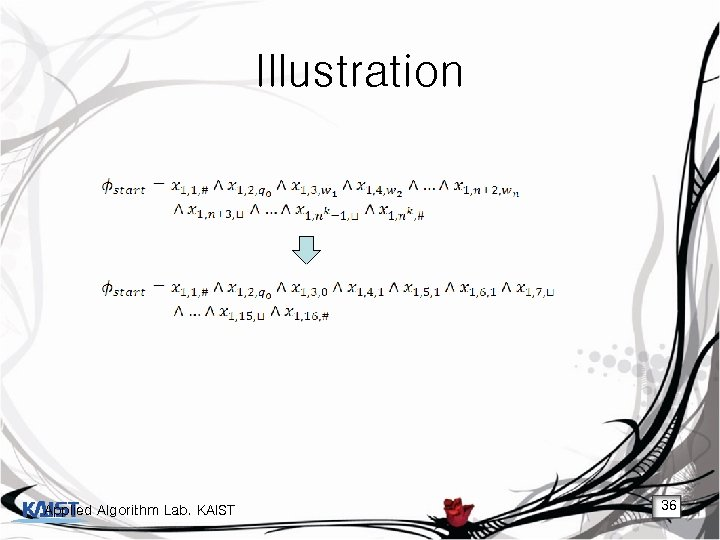Illustration Applied Algorithm Lab. KAIST 36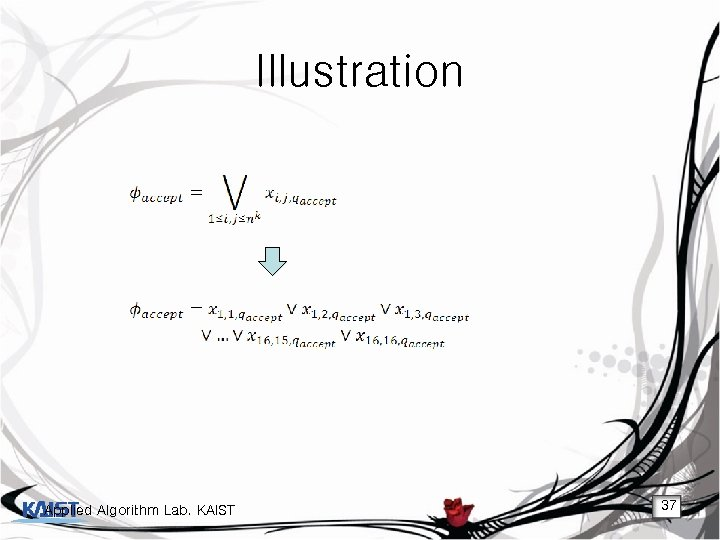Illustration Applied Algorithm Lab. KAIST 37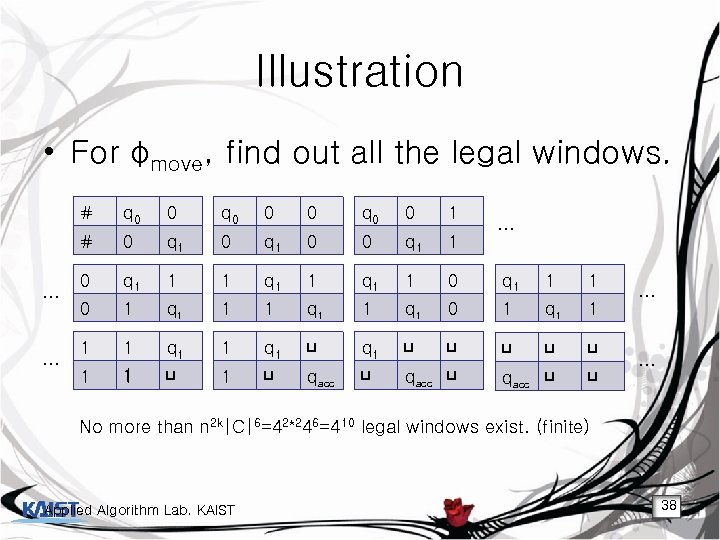Illustration • For φmove, find out all the legal windows. # … 0 q 0 0 1 # … q 0 0 q 1 1 1 q 1 1 0 q 1 1 1 0 1 q 1 1 q 1 1 q 1 ⊔ ⊔ ⊔ 1 1 ⊔ qacc ⊔ ⊔ … … … No more than n 2 k|C|6=42*246=410 legal windows exist. (finite) Applied Algorithm Lab. KAIST 38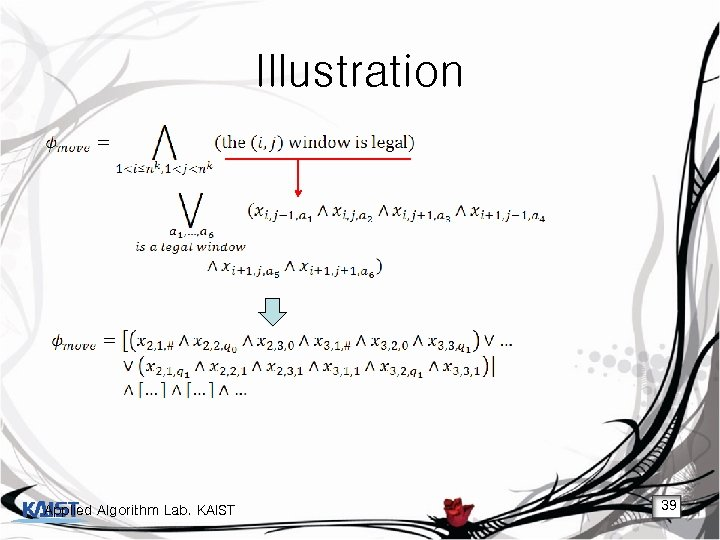Illustration Applied Algorithm Lab. KAIST 39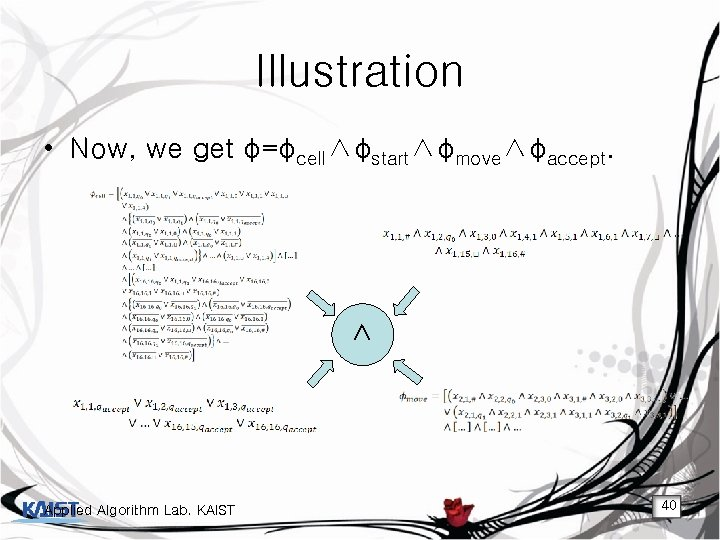Illustration • Now, we get φ=φcell∧φstart∧φmove∧φaccept. ∧ Applied Algorithm Lab. KAIST 40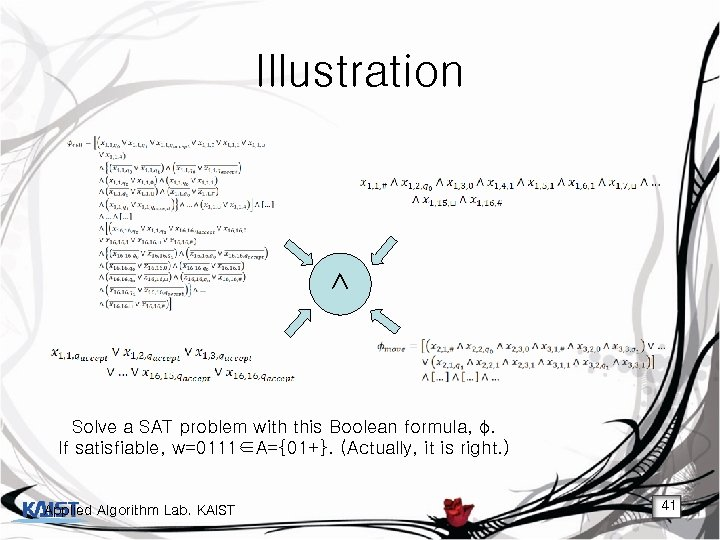Illustration ∧ Solve a SAT problem with this Boolean formula, φ. If satisfiable, w=0111∈A={01+}. (Actually, it is right. ) Applied Algorithm Lab. KAIST 41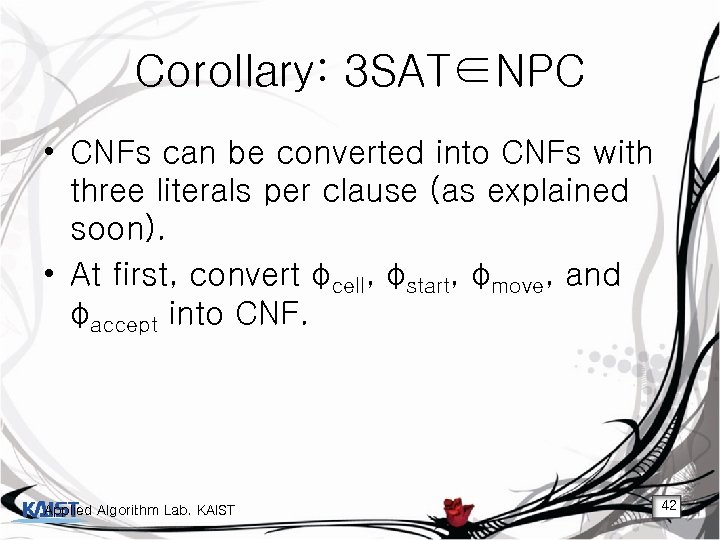Corollary: 3 SAT∈NPC • CNFs can be converted into CNFs with three literals per clause (as explained soon). • At first, convert φcell, φstart, φmove, and φaccept into CNF. Applied Algorithm Lab. KAIST 42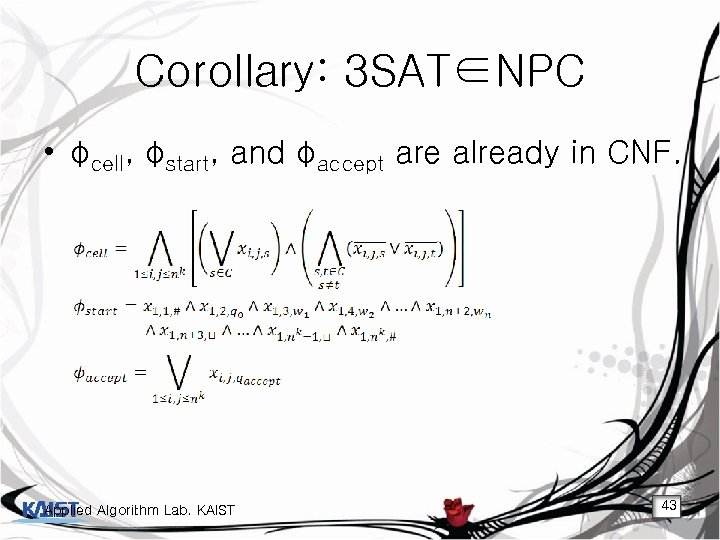Corollary: 3 SAT∈NPC • φcell, φstart, and φaccept are already in CNF. Applied Algorithm Lab. KAIST 43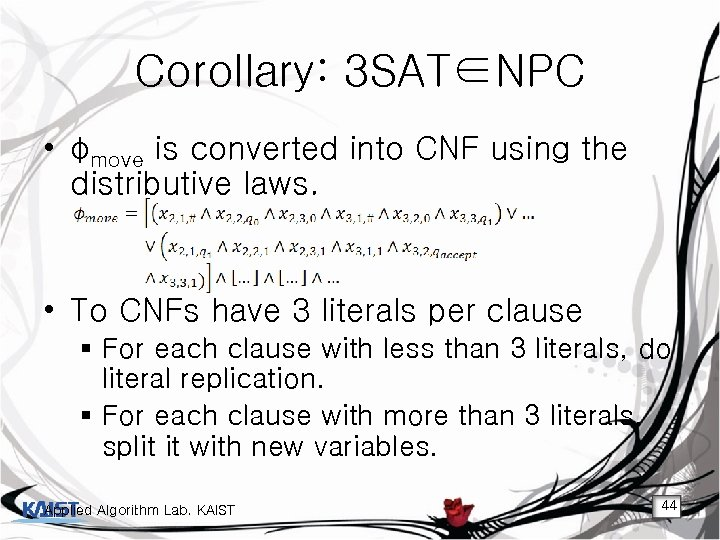Corollary: 3 SAT∈NPC • φmove is converted into CNF using the distributive laws. • To CNFs have 3 literals per clause § For each clause with less than 3 literals, do literal replication. § For each clause with more than 3 literals, split it with new variables. Applied Algorithm Lab. KAIST 44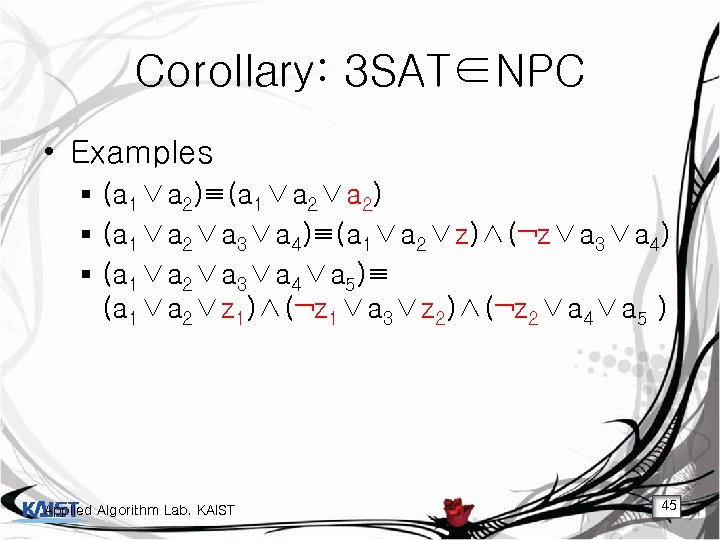Corollary: 3 SAT∈NPC • Examples § (a 1∨a 2)≡(a 1∨a 2) § (a 1∨a 2∨a 3∨a 4)≡(a 1∨a 2∨z)∧(¬z∨a 3∨a 4) § (a 1∨a 2∨a 3∨a 4∨a 5)≡ (a 1∨a 2∨z 1)∧(¬z 1∨a 3∨z 2)∧(¬z 2∨a 4∨a 5 ) Applied Algorithm Lab. KAIST 45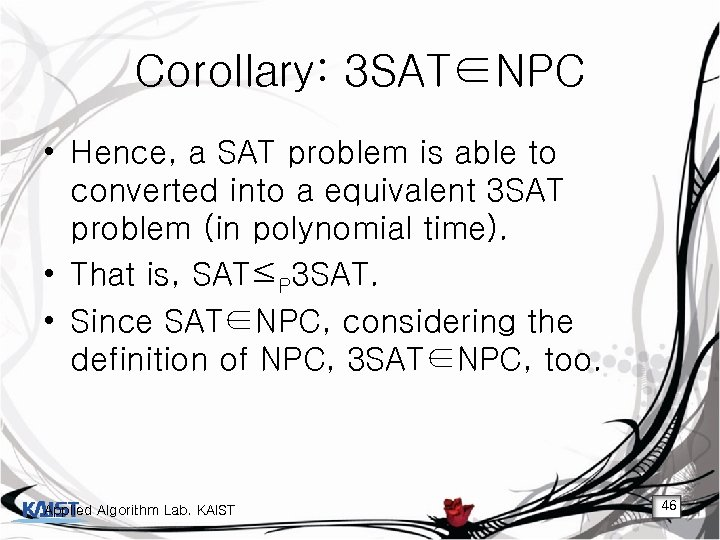Corollary: 3 SAT∈NPC • Hence, a SAT problem is able to converted into a equivalent 3 SAT problem (in polynomial time). • That is, SAT≤P 3 SAT. • Since SAT∈NPC, considering the definition of NPC, 3 SAT∈NPC, too. Applied Algorithm Lab. KAIST 46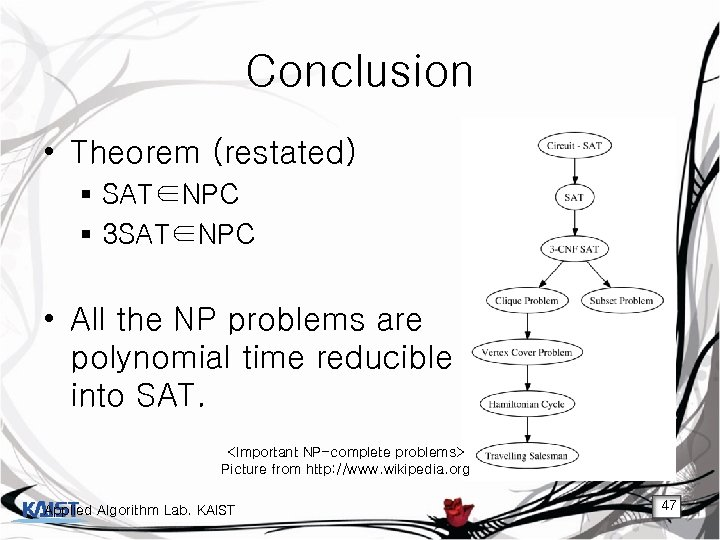Conclusion • Theorem (restated) § SAT∈NPC § 3 SAT∈NPC • All the NP problems are polynomial time reducible into SAT. Picture from http: //www. wikipedia. org Applied Algorithm Lab. KAIST 47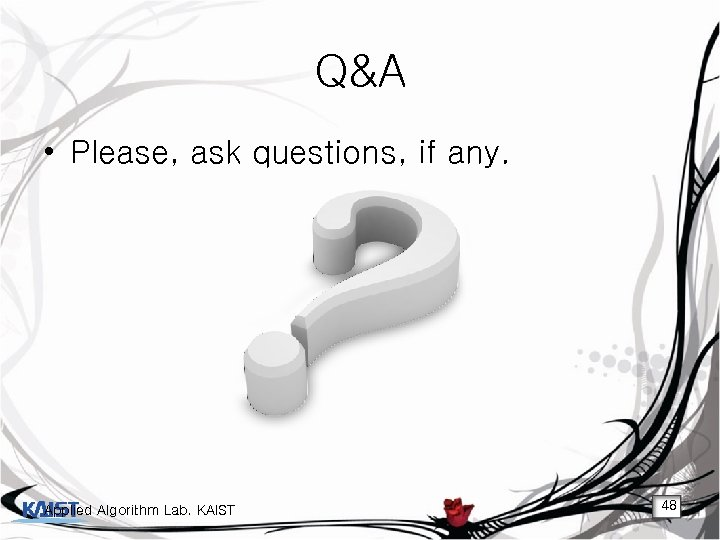Q&A • Please, ask questions, if any. Applied Algorithm Lab. KAIST 48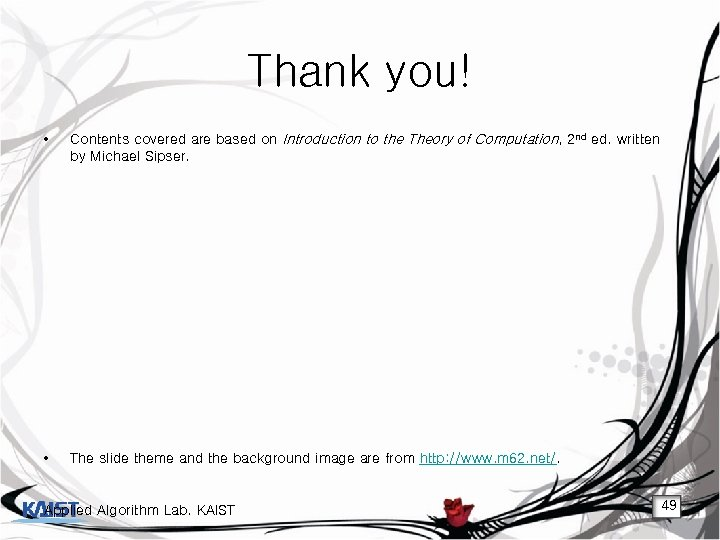Thank you! • Contents covered are based on Introduction to the Theory of Computation, 2 nd ed. written by Michael Sipser. • The slide theme and the background image are from http: //www. m 62. net/. Applied Algorithm Lab. KAIST 49